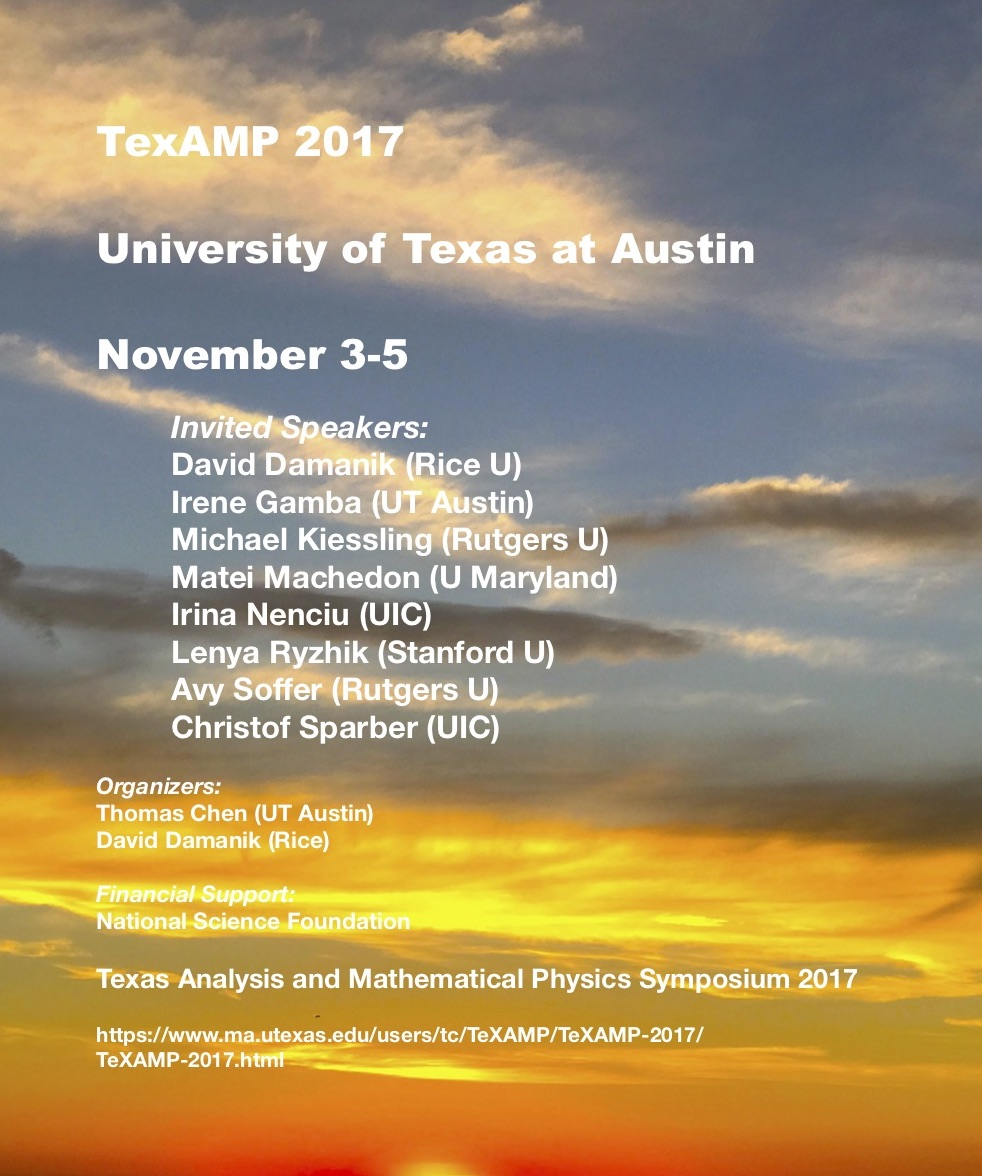# THE UNIVERSITY OF TEXAS AT AUSTIN### Abstracts

 Irene Gamba (University of Texas at Austin): Common features between the quantum Boltzmann equation cold regimes and the wave turbulence model for stratified flows Both the quantum Boltzmann equation for bosons at very low temperature and the model for wave turbulence theory for stratified flows, exhibit many similarities. Both represent statistical flow models given by integral forms in velocity or momenta space who lack some conservation properties, while keeping the gain-loss rates structure like the classical Boltzmann equation and have dissipative sources. However, they share the structure that allows for the construction of weak solutions in the space on continuous functions in time (and differentiable for positive times), with k-moments ($L^1_N$) in wave space. This is done using techniques of ODE's methods in Banach spaces by characterizing invariant bounded, convex, closed solutions subsets of integrable solutions with bounded moments in velocity space. This is work reflecting collaborations with Ricardo Alonso, Leslie Smith, and Minh Binh Tran. David Damanik (Rice University): Some surprises in the spectral theory of almost-periodic Schrodinger operators. We discuss spectral properties of almost periodic Schr\"odinger operators. In particular we explain two paradigms that have emerged in this study, how they are supported by heuristics and rigorous results, but also how they have been challenged recently. Michael Kiessling (Rutgers University): General relativity at the (sub-)atomic scale The idea that General Relativity (GR) may have something to say about the (sub-)atomic world is about as old as GR itself, but very few physicists have taken this idea seriously, and lamentably little is known rigorously. In this talk I use the problem  of the "general-relativistic Dirac spectrum of Hydrogen"  to convey the conceptual and technical issues one is up against. Then I survey pertinent results obtained over the past several years in collaboration with my colleague Shadi Tahvildar-Zadeh and some of our students and postdocs. Matei Machedon (University of Maryland): Pair excitation and the mean field approximation of interacting Bosons I We discuss a coupled system for the condensate function \phi and the pair excitation k, which provides a Fock space approximation for the evolution of  Bosons with interaction potential N^{3 \beta} v(N^{\beta} (x-y)). As \beta approaches 1, we expect this system to capture the transition from NLS to the Gross-Pitaevskii equations. Currently, we can only handle the case \beta < 2/3. Part I of this talk will discuss the Analysis background of the proof, and Part II the Mathematical Physics aspects. Irina Nenciu (University of Illinois, Chicago): On some concrete criteria for quantum and stochastic confinement In this talk we will present several recent results on criteria ensuring the confinement of a quantum or a stochastic particle to a bounded domain in $\mathbb{R}^n$. These criteria are given in terms of explicit growth and/or decay rates for the diffusion matrix and the drift potential close to the boundary of the domain. As an application of the general method, we will discuss several cases, including some where the background Riemannian manifold (induced by the diffusion matrix) is geodesically incomplete. These results are part of an ongoing joint project with G. Nenciu (IMAR, Bucharest, Romania). Lenya Ryzhik (Stanford University): The random heat equation in dimensions three and higher We consider the heat equation with a multiplicative Gaussian potential in dimensions three and higher. We show that the renormalized solution converges to the solution of a deterministic diffusion equation with an effective diffusivity. We also prove that the renormalized large scale random fluctuations are described by the Edwards-Wilkinson model, that is, the stochastic heat equation (SHE) with additive white noise, with an effective diffusion and an effective variance. This is a joint work with Yu Gu and Ofer Zeitouni. Avy Soffer (Rutgers University): Supercritical wave equations I will review the problem of global existence for dispersive equations, in particular, supercritical equations. These equations who play a fundamental role in science, have been, and remain a major challenge in the field of Partial Differential Equations. They come in various forms, derived from Geometry, General Relativity, Fluid Dynamics , Field Theory. I present a new approach to classify the asymptotic behavior of wave equations, supercritical and others, and construct global solutions with large initial data. I will then describe current extensions to Nonlinear Schroedinger Equations. Christof Sparber (University of Illinois, Chicago): Weakly nonlinear time-adiabatic theory We revisit the time-adiabatic theorem of quantum mechanics and show that it can be extended to weakly nonlinear situations, that is to nonlinear Schroedinger equations in which either the nonlinear coupling constant or, equivalently, the solution is asymptotically small. To this end, a notion of criticality is introduced at which the linear bound states stay adiabatically stable, but nonlinear effects start to show up at leading order in the form of a slowly varying nonlinear phase modulation. In addition, we prove that in the same regime a class of nonlinear bound states also stays adiabatically stable, at least in terms of spectral projections. Return to main page